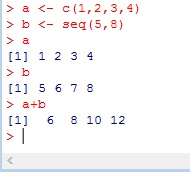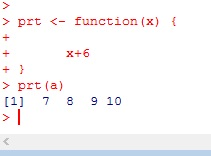# R: Vector operations

This is one area where R really shines. Consider two vectors a= (1,2,3,4) and b= (5,6,7,8). If you wanted to add the elements of the two vectors together like this:

1+5,2+6,3+7,4+8

In most programming languages, you would need to utilize a loop. And you could do it with a loop in R.

```for (i in 1:4){
print(a[i]+b[i])
}```

Or, thanks to vector operations, you can just use the + signYou can also use -, *, /You  can pass vector to a function and it will automatically iterate through it for you.